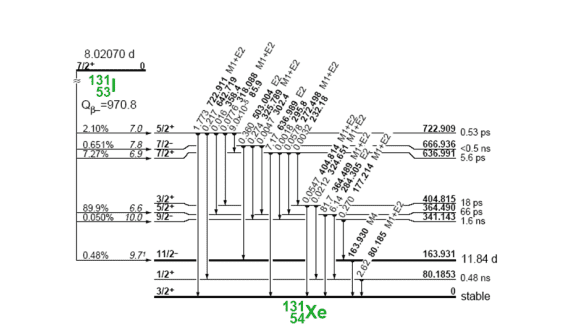Apologies for this question, but I'm trying to understand what I'm looking at here and was unable to find an answer online.From my understanding, this is a decay scheme that shows the percentage of each energy level at which the disintegrated mother nucleus has reached after the disintegration has occurred. But what do the other numbers and arrows show?

Where I'm stuck is the symbols and numbers. I've tried to look in my textbook as well to no avail.

I would really appreciate your help.

Many thanks!

#### Attachments

Last edited by a moderator:

mfb
Mentor
The half-integer values on the left with the signs are spin and parity. At the right side you have the energies (in keV) and lifetimes of the excited states.
The arrows are possible follow-up decays of xenon. Their labels: Not sure what the first number is. Partial decay width would be reasonable but doesn't fit well. The second number is the energy difference, the third one tells you which type of decay it is.

•Mahavir
gleem
Starting from left to right..The percentage under the I131 are the percentage of the beta decays that lead to the various energy levels of the daughter . The next number is the Log of the comparative half lives for the decays to the various levels. The comparative half live is proportional to the decay constant for that transition. The next number with the +/- signs are the spin and parity of the Xe131 states. The numbers above the energy levels in Xe131 are the percent of total gamma decay for that transition, the next above is the energy of the gamma ray for that transition, and the M1, E2, M4 refer to the type of electromagnetic transitions, magnetic dipole, electric quadrapole and magenetic octopole respectively. The numbers to the right of the Xe131 energy levels are the energies and half lives of those states. The Qβ- is he Q-value of the decay, the excess energy of the decay available to the beta particles as kinetic energy.

Edit: The M4 term should have been hexadecapole not octopole.

Last edited:
•Mahavir
Starting from left to right..The percentage under the I131 are the percentage of the beta decays that lead to the various energy levels of the daughter . The next number is the Log of the comparative half lives for the decays to the various levels. The comparative half live is proportional to the decay constant for that transition. The next number with the +/- signs are the spin and parity of the Xe131 states. The numbers above the energy levels in Xe131 are the percent of total gamma decay for that transition, the next above is the energy of the gamma ray for that transition, and the M1, E2, M4 refer to the type of electromagnetic transitions, magnetic dipole, electric quadrapole and magenetic octopole respectively. The numbers to the right of the Xe131 energy levels are the energies and half lives of those states. The Qβ- is he Q-value of the decay, the excess energy of the decay available to the beta particles as kinetic energy.

Edit: The M4 term should have been hexadecapole not octopole.
The half-integer values on the left with the signs are spin and parity. At the right side you have the energies (in keV) and lifetimes of the excited states.
The arrows are possible follow-up decays of xenon. Their labels: Not sure what the first number is. Partial decay width would be reasonable but doesn't fit well. The second number is the energy difference, the third one tells you which type of decay it is.

Thank you both ever so much! It makes a great deal more sense.